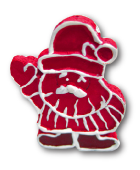### Challenge from 4. December

Christmas Candles

Authors: Cor Hurkens, Hennie ter Morsche

Project: 4TU.AMI

Challenge:

The packer-pixy Paco packs fifteen Christmas candles upright into a box with a square base. Each candle is shaped like a cylinder with a circle of radius 1 cm as its base. The picture shows the base of the box as a square and the bases of the fifteen candles as fifteen circles.The picture shows which candles touch each other and which candles touch the boundary of the box. The side length s of the square can be written in the form s = a+√b+√c(measured in centimeters) with three integers a, b, c.

What is the value of the sum a+b+c of these three integers?

Artwork: Frauke JansenPossible answers:

1. The sum of a, b, c is 8.

2. The sum of a, b, c is 9.

3. The sum of a, b, c is 10.

4. The sum of a, b, c is 11.

5. The sum of a, b, c is 12.

6. The sum of a, b, c is 13.

7. The sum of a, b, c is 14.

8. The sum of a, b, c is 15.

9. The sum of a, b, c is 16.

10. The sum of a, b, c is 17.

You have to log in to be able to submit your answer.